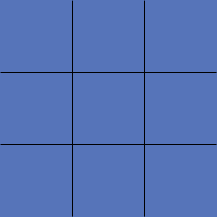SEARCH HOMEMath Central Quandaries & QueriesQuestion from TJ: 21 bags at 2 cubic feet per bag. How many yards would that be?TJ,

There are 3 feet in a yard.

If you want to cover an square region that is 1 yard by 1 yard with square tiles that are 1 foot by 1 foot it will take you 3 × 3 = 9 tiles. Thus there are 9 square feet in a square yard.If you want to fill a box that is 1 yard by 1 yard by 1 yard with boxes that are 1 foot by 1 foot by 1 foot it will take you 3 × 3 × 3 = 27 boxes. Thus there are 27 cubic feet in a cubic yard.

You have 21 bags each of which holds 21 cubic feet so you has 21 × 2 = 42 cubic feet of material. Since there are 27 cubic feet in a cubic yard that's 42/27 = 1.56 cubic yards.

I hope this helps,
PennyMath Central is supported by the University of Regina and The Pacific Institute for the Mathematical Sciences.